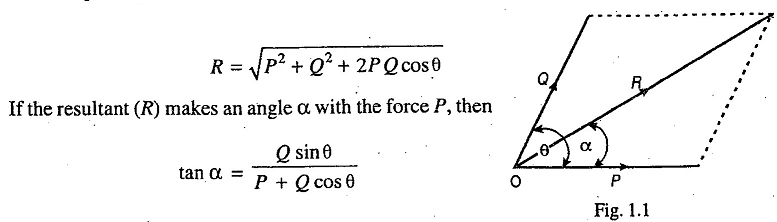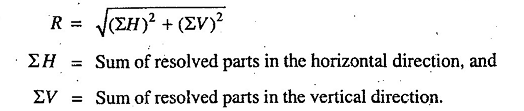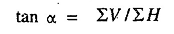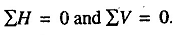### Resultant Force

Resultant Force - It is a single force which produces the same effect as produced by all the given forces acting on a body. The resultant force may be determined by the following three laws of forces :

1. Parallelogram law of forces. It states that if two forces, acting simultaneously on a particle, be represented in magnitude and direction by the two adjacent sides of a parallelogram, then their resultant may be represented in magnitude and direction by the diagonal of a parallelogram which passes through their points of intersection.

For example, let us consider two forces P and Q acting at angle θ at point O as shown in Fig. 1.1. The resultant is given by,2. Triangle law of forces. It states that if two forces, acting simultaneously on a particle, be represented in magnitude and direction by the two sides of a triangle taken in order, then their resultant may be represented in magnitude and direction by the third side of the triangle taken in opposite order.

3. Polygon law of forces. It states that if a number of forces, acting simultaneously on a particle, be represented in magnitude and direction by sides of a polygon taken in order, then their resultant is represented in magnitude and direction by the closing side of the polygon taken in opposite order.

Notes :
1. The resultant of more than two intersecting forces may be found out by resolving all the forces horizontally and vertically. In such cases, resultant of the forces is given byIf the resultant (R) makes an angle α with the horizontal, then2. If the resultant of a number of forces, acting on a particle, is zero then the particle will be in equilibrium. Such a set of forces, whose resultant is zero, are known as equilibrium forces. The force, which brings the set of forces in equilibrium is called an equilibriant. It is equal to the resultant force in magnitude but opposite in direction.

3. A number of forces acting on a particle will be in equilibrium when:1.